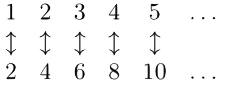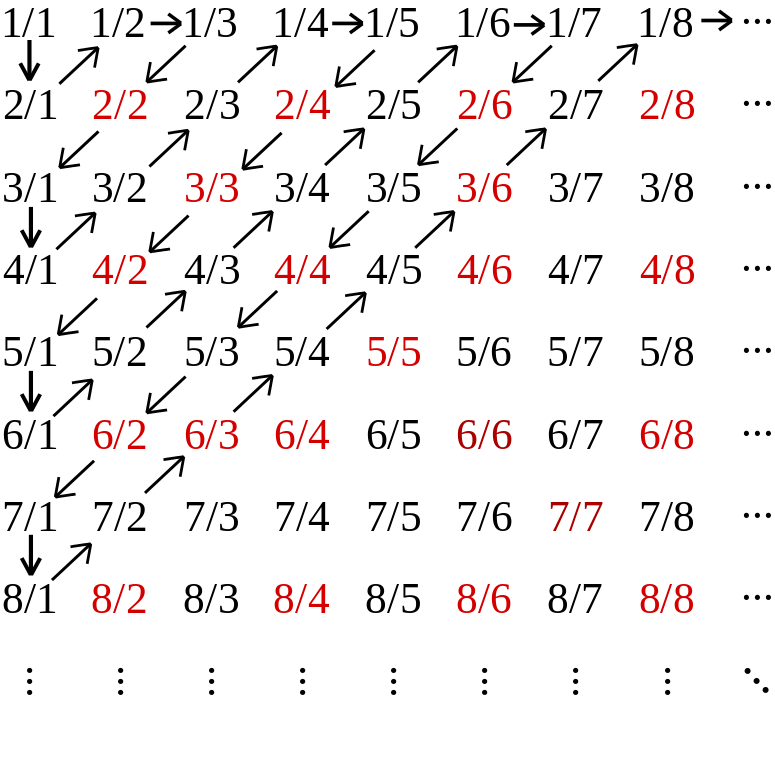Dodgeball
A game that teaches you something amazing about infinity!

The rules of Dodgeball

The game below is played between two players, a Matcher and a Dodger. Each player has their own grid of tiles, and repeated clicks on a tile toggle its state between X and O.

Each turn the Dodger turns a single tile, and the Matcher makes a complete row. The turn is finished when the ↩ button is pressed on the Matcher's grid. The tiles from that turn are then locked, and new tiles appear.

After all turns are complete, the Dodger has a single row of Xs and Os, and the Matcher has several rows. If any one of the Matcher's rows matches the Dodger's row, the Matcher wins. Otherwise the Dodger wins. Click the ? mark button to check.

Now let's play

Choose a size for the game, and play as either two players, or get the computer to be the Matcher by hitting the Automatch button.

Player 1 — The Matcher

Player 2 — The Dodger

So what has this got to do with infinity?

Have you mastered the game? If you have, you will know that the Dodger should always win. If not, keep at it, and read the below when you've succeeded.

Click here for a discussion of the implications of Dodgeball for infinity.Georg Cantor (1845-1918)
Towards the end of the 19th century, the German mathematiciam Georg Cantor published some results that completely changed the way infinity was understood. They generated a great deal of controversy, but the basic arguments are both simple and beautiful.

Central to his argument is the idea of the size of a set, and, in particular, what it means to say that two sets are the same size. Cantor said that two sets are the same size if they can be put in one-to-one correspondence, that is:

If every element of one set can be matched to a unique element of another, such that no elements in either set remain unmatched, then those two sets are the same size.

For everyday, finite sets, this is a simple and intuitive notion. For example, if you are laying a table and every bowl has a spoon next to it, you know you have the same number of bowls as spoons — there is no need to count them and check.

Where things get interesting is when the sets are infinite. For example, consider the set of all positive integers {1, 2, 3, … } and the set of all even positive integers {2, 4, 6, … }. It would seem that the first set is larger, but since any element n in the first set can be matched uniquely to 2n in the second, we can actually show that both sets are the same size.What about the rationals? It turns out that the rationals can also be put into one-to-one correspondence with the positive integers by following the arrows in the following diagram:But what about all real numbers — i.e. all decimals, including irrationals like π and √2? Cantor imagined an infinitely long list containing all the decimals between 0 and 1. Below is an example, but the values can be in any order.

0.60801298334263…
0.01949042174951…
0.05714191822315…
0.96473069139756…
0.77767780516297…
0.92023350438103…
0.39258143678308…
0.67825716058724…
0.83253744337708…
0.21415415313095…
Writing out this list is like playing the Matcher in an infinite version of Dodgeball with the numbers after the decimal point. We have seen that Dodger always wins the finite version — is this still the case when the game is infinite?

If the Dodger can indeed still win, then we have found a number between 0 and 1 that isn't in the list, contradicting the statement that the list had all the numbers between 0 and 1. This means that it is impossible to make any such list, and we have shown, incredibly, that:

Even though the number of integers is infinite, the number of real numbers is larger. We have found a number larger than infinity!

Can you use the Dodger's strategy to find the first 10 digits of a number that cannot be in the example list?

Hide the discussion.

Diagonal argument image by Cronholm144 (https://commons.wikimedia.org/wiki/File:Diagonal_argument.svg)